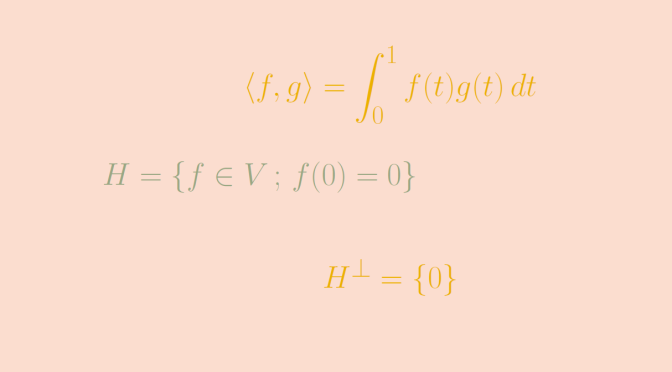# A proper subspace without an orthogonal complement

We consider an inner product space $$V$$ over the field of real numbers $$\mathbb R$$. The inner product is denoted by $$\langle \cdot , \cdot \rangle$$.

When $$V$$ is a finite dimensional space, every proper subspace $$F \subset V$$ has an orthogonal complement $$F^\perp$$ with $$V = F \oplus F^\perp$$. This is no more true for infinite dimensional spaces and we present here an example.

Consider the space $$V=\mathcal C([0,1],\mathbb R)$$ of the continuous real functions defined on the segment $$[0,1]$$. The bilinear map
$\begin{array}{l|rcl} \langle \cdot , \cdot \rangle : & V \times V & \longrightarrow & \mathbb R \\ & (f,g) & \longmapsto & \langle f , g \rangle = \displaystyle \int_0^1 f(t)g(t) \, dt \end{array}$ is an inner product on $$V$$.

Let’s consider the proper subspace $$H = \{f \in V \, ; \, f(0)=0\}$$. $$H$$ is an hyperplane of $$V$$ as $$H$$ is the kernel of the linear form $$\varphi : f \mapsto f(0)$$ defined on $$V$$. $$H$$ is a proper subspace as $$\varphi$$ is not always vanishing. Let’s prove that $$H^\perp = \{0\}$$.

Take $$g \in H^\perp$$. By definition of $$H^\perp$$ we have $$\int_0^1 f(t) g(t) \, dt = 0$$ for all $$f \in H$$. In particular the function $$h : t \mapsto t g(t)$$ belongs to $$H$$. Hence
$0 = \langle h , g \rangle = \displaystyle \int_0^1 t g(t)g(t) \, dt$ The map $$t \mapsto t g^2(t)$$ is continuous, non-negative on $$[0,1]$$ and its integral on this segment vanishes. Hence $$t g^2(t)$$ is always vanishing on $$[0,1]$$, and $$g$$ is always vanishing on $$(0,1]$$. As $$g$$ is continuous, we finally get that $$g = 0$$.

$$H$$ doesn’t have an orthogonal complement.

Moreover we have
$(H^\perp)^\perp = \{0\}^\perp = V \neq H$